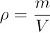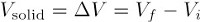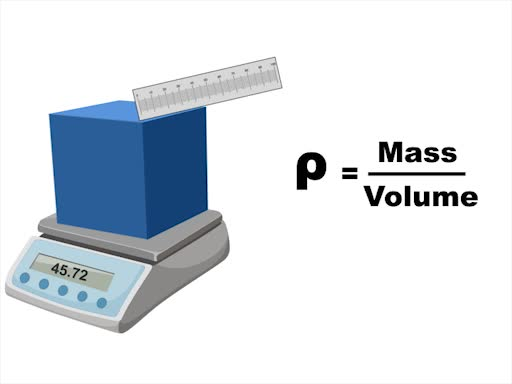Trial ends in

# Determining the Density of a Solid and Liquid

### Overview

Source: Laboratory of Dr. Michael Evans — Georgia Institute of Technology

The ratio of the mass of a substance to its volume is known as the mass density or, simply, the density of the substance. Density is expressed in units of mass per volume, such as g/mL or kg/m3. Because the density of a substance does not depend on the amount of substance present, density is an “intensive property”.

To measure the density of a sample of material, both the mass and volume of the sample must be determined. For both solids and liquids, a balance can be used to measure mass; however, methods for determining volume are different for solids and liquids. As liquids can flow and take the shapes of their containers, glassware such as a graduated cylinder or volumetric flask can be used to measure the volume of a liquid. The volume of an irregularly-shaped solid can be measured by submersion in a liquid — the difference in volume caused by addition of the solid is equal to the volume of the solid.

This demonstration illustrates the methods for measuring the density of solids and liquids. Using a volumetric flask and an analytical balance, the density of ethanol can be determined. Using a graduated cylinder, analytical balance, and water as the displaced liquid, the density of zinc metal can be determined.

### Principles

By definition, all matter has mass and occupies volume. The density of a substance is the ratio of its mass to its volume. At constant temperature and pressure, the density of a substance is constant.Density is an intensive property of a substance that doesn’t depend on the amount of substance present. Thus, density can be used to identify an unknown pure substance if a list of reference densities is available, and the experimenter can choose a convenient amount of substance to work with when measuring density.

To measure the density of a sample of a substance, it is necessary to measure its mass and volume. Mass is typically measured using an analytical balance, a precise instrument that relies on the force exerted by the sample due to gravity. The container to hold the sample (also used to measure volume) is weighed and tared, so only the sample mass appears on the balance display when the sample is added to the container.

For liquids, this container is typically a volumetric flask, which has one marking that corresponds to a specific volume. The container is filled to the line with the liquid sample and weighed again after the empty flask has been tared. The measured density is the ratio of the measured mass to the volume indicated on the flask.

Most solid substances are irregularly shaped, which complicates volume determination. It is inaccurate, for example, to determine the volume of a powder by measuring its dimensions. Instead of directly measuring dimensions or using glassware like a volumetric flask, it is necessary to make use of a liquid displacement method to measure the volume of an irregularly shaped solid. A graduated cylinder containing a known volume of liquid (in which the solid is insoluble) is tared. The solid is added to the cylinder, and the total mass is weighed again to determine the mass of the solid. Addition of the solid causes an upward displacement of the liquid, resulting in a new volume reading. The volume of the solid is equal to the change in volume due to liquid displacement (i.e., the difference in liquid volume before and after adding solid).As for liquids, the measured density of a solid sample is the ratio of the measured mass to the measured volume.

### Procedure

1. Determination of the Density of Liquid Ethanol

1. Place a clean and dry 50-mL volumetric flask on an analytical balance.
2. Press the “Tare” or “Zero” button on the balance. The balance should read 0.000 g.
3. Use a buret funnel to add 45 mL of liquid ethanol to the volumetric flask.
4. Use a Pasteur pipette to add the final 5 mL of liquid, just until the bottom of the liquid’s meniscus touches the marking on the flask.
5. Weigh the volumetric flask again and record the mass of the ethanol.
6. For best results, repeat steps 1.1 – 1.5 twice more to obtain two additional density measurements.

2. Determination of the Density of Solid Zinc Metal

1. Add approximately 40 mL of water to a clean and dry 100-mL graduated cylinder. Record the exact volume of the water.
2. Place the cylinder and water on an analytical balance. Press the “Tare” or “Zero” button on the balance. The balance should read 0.000 g.
3. Add approximately 10 zinc pellets to the graduated cylinder. Record the new volume of the water plus zinc pellets using the liquid level after addition of the zinc (Figure 1).Figure 1. Zinc added to the cylinder on the right causes the water level to be displaced upward.
4. Weigh the cylinder, water, and zinc pellets on the balance. Record the mass of the zinc pellets.
5. For best results, repeat steps 2.1 – 2.4 twice more to obtain two additional density measurements.

Density, defined as a substance's mass per unit volume, is an important physical property for characterizing a material or chemical system.

Mathematically, density is calculated as a substance’s mass per the volume it occupies. The Greek symbol "ρ" is normally used to denote density in the physical sciences. To obtain the density of a substance, its mass and volume are determined by measurement.

This video will introduce the principles of density determination, the procedures for calculating the density of both solid and liquid substances, and some applications of density in scientific research.

All matter has mass, and that mass occupies a specific volume.

However, the volume of space occupied by the same mass is different for different substances, depending on their respective density. For example, a ton of bricks has the same mass as a ton of feathers, but occupies considerably less volume. Density is obtained by dividing mass by volume. . Mass can be measured with scales or balances, and is expressed in grams or kilograms.

By convention, the volume of liquids and gases is often expressed in units of liters or milliliters, measured with glassware. The dimensions of regularly shaped solids can be measured directly with rulers or calipers, which have linear units, giving volumes in units such as cubic centimeters. One milliliter is equivalent to one cubic centimeter.

The dimensions of irregularly shaped solid samples cannot be easily measured. Instead, their volumes can be determined by submerging the solid in a liquid. The volume of the submerged solid is equal to the volume of liquid displaced.

Now that you understand the concept of density, let's take a look at two protocols for accurately determining the density of a liquid and a solid.

To begin this procedure, place a clean and dry 50-mL volumetric flask on an analytical balance. After the measurement has stabilized, tare the balance. The balance should read zero. Use a funnel to add approximately 45 mL of liquid to the flask. Do not fill to the calibration mark. Use a Pasteur pipette to carefully add the final 5 mL of liquid, just until the bottom of the liquid's meniscus touches the line on the flask. Weigh the flask again and record the mass of the liquid. Repeat the measurements at least twice to obtain additional values to calculate an average density. The results are shown in this table. The average measured density was 0.789 g/mL, matching the literature value for ethanol.

To determine the density of an irregular solid in pellet form, add approximately 40 mL of water to a clean and dry 100-mL graduated cylinder. Record the exact volume. Place the cylinder on an analytical balance and tare. Add approximately 10 pellets, and record the new volume after the addition. Weigh the cylinder, water, and pellets. The mass is only the pellets, as the rest have been tared. Make at least two additional sets of mass and volume measurements to calculate an average value of the density. The density for zinc was measured for three different samples. It was found to be 6.3 g/mL. Note that, since the measurements were made in a graduated cylinder, which is less precise than a volumetric flask, the density has lower degree of precision.

Let's now look at several different applications of density to different field of scientific research.

Density is useful for identifying or validating pure materials, such as elements or other species of known purity. For example, because gold has a higher density than many other cheaper metals, calculating the density of a gold coin is a quick and inexpensive way to test for its purity. If the density does not match that of gold, the coin is not pure. Here, a gold coin was found to have a mass of 27.55 g and a volume of 1.84 cm3, giving a density of 14.97 g/cm3, which is significantly less than gold's density of 19.3 g/cm3, indicating that the coin is not made of pure gold.

Density measurements can also be used to identify an unknown substance if a list of possible reference densities is available, and can be used to distinguish between metals similar in appearance. In this example, the scientist is trying to identify two samples of shiny silver metal, which could be either aluminum or zinc. While the two samples have the same mass, their volumes are considerably different. The densities were determined to be 2.7 and 7.1 g/cm3 respectively, confirming their identities as aluminum and zinc.

Finally, differences in density are useful for separating components of a complex mixture. In a method called density gradient centrifugation, decreasing concentrations of sucrose or polymers are layered to create a gradient. The sample is then added on top. This mixture is then subjected to centrifugation — the spinning of the mixture at high speed to generate a "centrifugal force" that will lead to the formation of a concentration gradient of the molecule. Components of the mixture will migrate to a point along this gradient with which its density is comparable.

In this example, a specific type of lipid droplets, or small drops of fat molecules, was isolated from cells. A homogenized mixture was first obtained by breaking the cells open. By centrifuging the mixture in a sucrose density gradient, the droplets were successfully separated from other cellular components that are made of lipids, such as the cells’ membranes.

You've just watched JoVE's introduction the determination of the density of a liquid and a solid. You should now understand mass, volume, and density, as well as having a good idea of how to measure these quantities.

Thanks for watching!

### Results

Table 1 lists results for the determination of the density of ethanol using a 50-mL volumetric flask. Densities were calculated by dividing the measured mass by 50.0 mL. The mean measured density was 0.789 ± 0.001 g/mL. Table 2 lists results for the determination of the density of a sample of zinc metal using a 100-mL graduated cylinder and the liquid displacement method. Note that the measured densities are constant (within experimental error) for both substances. Table 2, in particular, demonstrates that density is independent of the amount of substance studied.

 Trial Mass of Ethanol (g) Volume of Ethanol (mL) Density (g/mL) 1 39.448 50.0 0.789 2 39.392 50.0 0.788 3 39.489 50.0 0.790

Table 1. Results for the determination of the density of ethanol using a 50-mL volumetric flask.

 Trial Mass of Zinc (g) Volume of Zinc (mL) Density (g/mL) 1 5.6133 0.9 6.2 2 7.6491 1.2 6.3 3 8.2164 1.3 6.3

Table 2. Results for the determination of the density of a sample of zinc metal using a 100-mL graduated cylinder and the liquid displacement method.

### Applications and Summary

Density is a characteristic intensive property of a substance. Thus, density measurements can be used to identify an unknown pure substance if a list of possible reference densities is available. For example, density can be used to distinguish between metals similar in outward appearance (Figure 2).

In contexts where very low or very high mass is desirable, density is a critical material property. Materials engineers carefully consider the density of materials for construction in these contexts. For example, the bodies of some lightweight laptop computers are made of aluminum, one of the least dense metals. Lightweight tennis rackets contain titanium, another low-density metal.Figure 2: Equivalent masses of aluminum (Al) and zinc (Zn) metal. The zinc metal occupies a much smaller volume due to its higher density.Play Video

### Cite this

JoVE Science Education Database. General Chemistry. Determining the Density of a Solid and Liquid. JoVE, Cambridge, MA, (2021).More

JoVE Science Education Database. General Chemistry. Determining the Density of a Solid and Liquid. JoVE, Cambridge, MA, (2021).

Less
Copy Citation
View Video

### Get cutting-edge science videos from JoVE sent straight to your inbox every month.X